# MCQs on Electric Circuits

##### Page 5 of 63. Go to page 1 2 3 4 5 6 7 8 9 10 11 12 13 14 15 16 17 18 19 20 21 22 23 24 25 26 27 28 29 30 31 32 33 34 35 36 37 38 39 40 41 42 43 44 45 46 47 48 49 50 51 52 53 54 55 56 57 58 59 60 61 62 63
01․ If a resistor is connected across the voltage source and the frequency of voltage and current wave form is 50Hz, then what is frequency of instantaneous power
0 Hz.
100 Hz.
50 Hz.
150 Hz.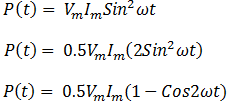Therefore, frequency is doubled for the instantaneous power.

02․ If a pure inductor is connected across the ac source, the average power taken by the inductor is
a few watt.
100 watt.
zero watt.
maximum power.

In the positive half cycle of power waveform ideal inductor takes energy from the source. In the negative half cycle of power waveform ideal inductor delivers the power to the source. Therefore net power taken by the ideal inductor is zero.

03․ Average power taken by the pure capacitor is
Zero
Minimum
Maximum
Any of the above

In positive half cycle charging occurs and in negative half cycle discharging will occur. So net power for an ideal capacitor over a full cycle is zero.

04․ In a series R, L circuit, voltage across resistor and inductor are 3 V and 4 V respectively, then what is the applied voltage?
7V
5V
4V
3V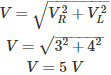05․ The alternative names for active power is/are
real power.
average power.
true power.
all of the above.

Different names for active power are
• Real power
• Average power
• True power
• Net power
These all are equal to VIcosθ.

06․ In series R, L circuit power factor can be defined as
R/Z
P/S
Vr/V
All of the above

These are all the different forms of representing power factor for R, L series circuit. Mathematically power factor factor can be defined as cosine of angle between voltage phasor and current phasor. By using the voltage triangle, power triangle and impedance triangle,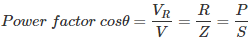07․ In ac RC series circuit total voltage is 10V and voltage across resistor is 6V, then what is voltage across capacitor?
4V
8V
16V
10V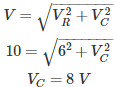Therefore the voltage across capacitor is 8 V.

08․ In series RLC circuit, voltage across resistor, inductor and capacitor are 5V, 2V and 2V respectively. Find total voltage?
9V
4V
2V
5V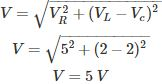09․ In RLC series circuit, if the voltage across capacitor is greater than voltage across inductor, then power factor of the network is
lagging.
unity.
zero.

Voltage across capacitor is more than inductor means, capacitive reactance of the network is more than inductive reactance. Therefore the power factor is leading.

10․ In RLC parallel circuit current through resistor, inductor and capacitor are 10A, 5A and 5A respectively. What is the total current in the circuit?
20A
10A
5A
0A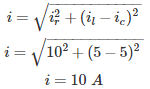<<<34567>>>